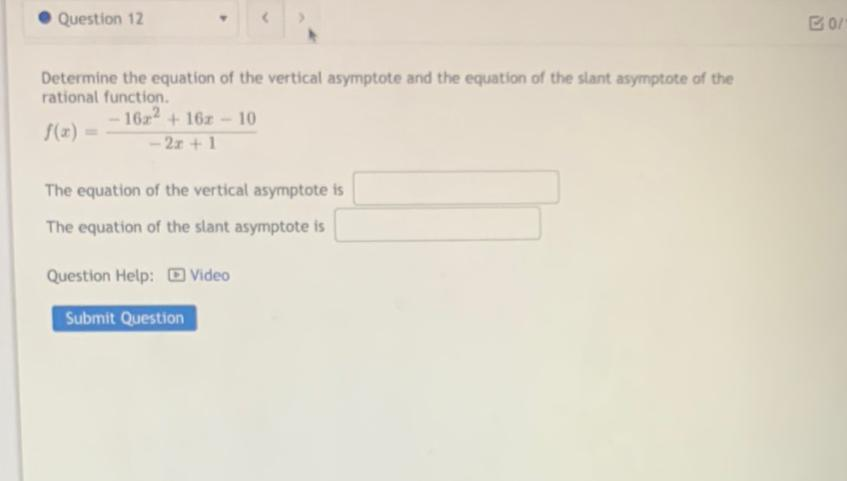Question:

# Question 12 B01 Determine the equation of the vertical asymptote and the equation of the slant asymptote of the rational functioQuestion 12 B01 Determine the equation of the vertical asymptote and the equation of the slant asymptote of the rational function. - 16x2 + 162 - 10 f(x) = - 2x+1 The equation of the vertical asymptote is The equation of the slant asymptote is Question Help: Video Submit Question